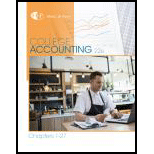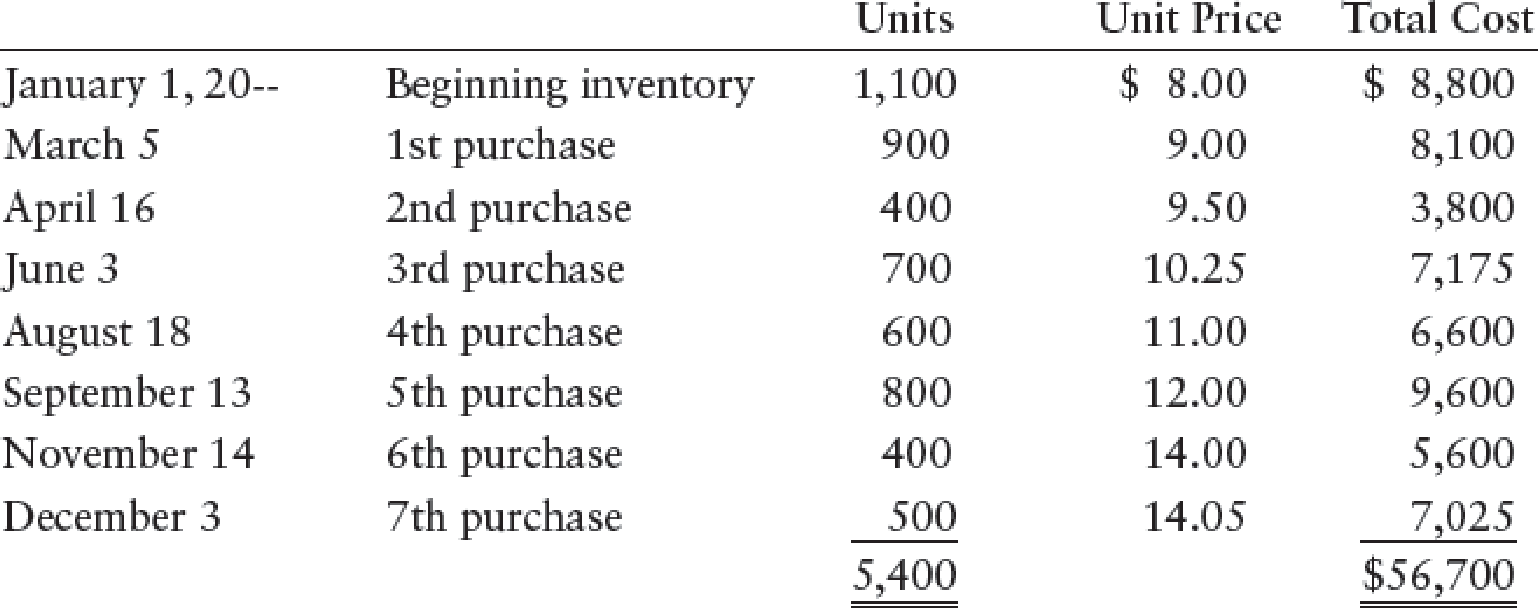# COST ALLOCATION AND LOWER-OF-COST-OR-MARKET Douglas Company’s beginning inventory and purchases during the fiscal year ended December 31, 20--, were as follows: There are 1,000 units of inventory on hand on December 31. REQUIRED 1. Calculate the total amount to be assigned to the ending inventory and cost of goods sold on December 31 under each of the following methods: (a) FIFO (b) LIFO (c) Weighted-average (round calculations to two decimal places) 2. Assume that the market price per unit (cost to replace) of Douglas’s inventory on December 31 was $13. Calculate the total amount to be assigned to the ending inventory on December 31 under each of the following methods: (a) FIFO lower-of-cost-or-market (b) Weighted-average lower-of-cost-or-market 3. Prepare required entries to apply: (a) FIFO lower-of-cost-or-market (b) Weighted-average lower-of-cost-or-marketBuyFindarrow_forward ### College Accounting, Chapters 1-27 ... 22nd Edition James A. Heintz + 1 other Publisher: Cengage Learning ISBN: 9781305666160 #### Solutions Chapter SectionBuyFindarrow_forward ### College Accounting, Chapters 1-27 ... 22nd Edition James A. Heintz + 1 other Publisher: Cengage Learning ISBN: 9781305666160 Chapter 13, Problem 7SPA Textbook Problem 162 views ## COST ALLOCATION AND LOWER-OF-COST-OR-MARKET Douglas Company’s beginning inventory and purchases during the fiscal year ended December 31, 20--, were as follows:There are 1,000 units of inventory on hand on December 31.REQUIRED 1. Calculate the total amount to be assigned to the ending inventory and cost of goods sold on December 31 under each of the following methods: (a) FIFO (b) LIFO (c) Weighted-average (round calculations to two decimal places) 2. Assume that the market price per unit (cost to replace) of Douglas’s inventory on December 31 was$13. Calculate the total amount to be assigned to the ending inventory on December 31 under each of the following methods: (a) FIFO lower-of-cost-or-market (b) Weighted-average lower-of-cost-or-market 3. Prepare required entries to apply: (a) FIFO lower-of-cost-or-market (b) Weighted-average lower-of-cost-or-market

1.

(a)

To determine

Calculate the total amount of cost of goods sold and cost of ending inventory on September 30, 20-2 under FIFO method.

### Explanation of Solution

First-in-First-Out (FIFO): In First-in-First-Out method, the first purchased items are sold first. The value of the ending inventory consists of the recently purchased items.

Calculate the total amount of cost of goods sold an...

2.

(b)

To determine

Calculate the total amount of cost of goods sold and cost of ending inventory on September 30, 20-2 under LIFO method.

3.

(c)

To determine

Calculate the total amount of cost of goods sold and cost of ending inventory on September 30, 20-2 under weighted average cost method.

2.

(a)

To determine

Calculate the cost of ending inventory under FIFO method (Lower of cost or market).

2.

(b)

To determine

Calculate the cost of ending inventory under weighted average cost method (Lower of cost or market).

3.

(a)

To determine

Show the journal entry would be made under lower-of-cost-or-market.

3.

(b)

To determine

Show the journal entry would be made under lower-of-cost-or-market.

### Still sussing out bartleby?

Check out a sample textbook solution.

See a sample solution

#### The Solution to Your Study Problems

Bartleby provides explanations to thousands of textbook problems written by our experts, many with advanced degrees!

Get Started